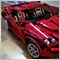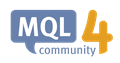# Script to select certain orders and then close them MT425

Hi I'm having trouble to select the first and last order that is opened in each currency pair and then closing them when reaching a certain profit both of them. Im trading a certain strategy that if the first order and last I want them to close first. so if I have 3 trades open, I want the first and third to close together. But Im having trouble closing them in my EA. Can you see what I am missing in my code below?

``` //GET VALUES
for (i = 0 ; i < OrdersTotal() ; i = i + 1)
{
if ( !(OrderSelect(i,SELECT_BY_POS,MODE_TRADES)) || OrderSymbol() != Symbol() || OrderMagicNumber() != MagicCurrentSelectOrderVar )   continue;

if(i == 1)
{
profitFirstOrder = OrderProfit() - OrderCommission();
}

if(i == OrdersTotal())
{
profitLastOrder = OrderProfit() - OrderCommission();
}
}

if(profitFirstOrder + profitLastOrder > TakeProfit)
{
closeFirstAndLastOrder = 1;
}

//CLOSER

for (i = 0 ; i < OrdersTotal() ; i = i + 1)
{
if ( !(OrderSelect(i,SELECT_BY_POS,MODE_TRADES)) || OrderSymbol() != Symbol() || OrderMagicNumber() != MagicCurrentSelectOrderVar )   continue;

if ( OrderType() == 0 && closeFirstAndLastOrder == 1 && OrdersTotal() > 1 && (i == 1 || i == OrdersTotal()))
{
if ( OrderClose(OrderTicket(),OrderLots(),Bid,3,Pink) )   return;
Print("OrderClose error ",GetLastError());
return;
}
if ( OrderType() == 1 && closeFirstAndLastOrder == 1 && OrdersTotal() > 1 && (i == 1 || i == OrdersTotal()))
{
Print("OrderClose error ",GetLastError());
return;
}
}```1749

Cleasfifthy:

Hi I'm having trouble to select the first and last order that is opened in each currency pair and then closing them when reaching a certain profit both of them. Im trading a certain strategy that if the first order and last I want them to close first. so if I have 3 trades open, I want the first and third to close together. But Im having trouble closing them in my EA. Can you see what I am missing in my code below?

Two issues:

(1) When you have this:

```for (i=0; i<OrdersTotal(); i++)
```

Your i will range from 0 to OrdersTotal()-1, it will never be equal to OrdersTotal(). So when you use i==1 and i==OrdersTotal() to determine first and last order, you'll never get what you want.

(2) Check out this page https://docs.mql4.com/trading/orderselect, which says that "Consecutive selection of orders using the SELECT_BY_POS parameter returns information in the sequence in which it was received from the trading server. Sorting of the resulting list of orders cannot be guaranteed." So you cannot use the value of your i to assume the opening sequence of your orders. Should always check the order open time.OrderSelect - Trade Functions - MQL4 Reference
• docs.mql4.com
To find out from what list the order has been selected, its close time must be analyzed. If the order close time equals to 0, the order is open or pending and taken from the terminal open orders list. One can distinguish an opened order from a pending order by the order type. If the order close time does not equal to 0, the order is a closed...Moderator
24771

This may give you some ideas

```#include <stdlib.mqh>

//+------------------------------------------------------------------+
void CheckFirstAndLast()
{
int firstTicket=0;
int latestTicket=0;

for(int x=OrdersTotal()-1;x>=0;x--)
{
if(OrderSelect(x,SELECT_BY_POS) && OrderSymbol()==_Symbol && OrderMagicNumber()==MagicCurrentSelectOrderVar)
{
if(OrderType()==OP_BUY || OrderType()==OP_SELL) //So that you won't be including pending orders
{
{
firstTicket=OrderTicket();
}
{
latestTicket=OrderTicket();
}
}
}
}
{
if(OrderSelect(firstTicket,SELECT_BY_TICKET))
{
if(!OrderClose(OrderTicket(),OrderLots(),OrderClosePrice(),3,clrPink))
Print(TimeCurrent()," Error with Closing Order #"+(string)OrderTicket()+ErrorDescription(GetLastError()));
}
if(OrderSelect(latestTicket,SELECT_BY_TICKET))
{
if(!OrderClose(OrderTicket(),OrderLots(),OrderClosePrice(),3,clrPink))
Print(TimeCurrent()," Error with Closing Order #"+(string)OrderTicket()+ErrorDescription(GetLastError()));
}
}
}
//+------------------------------------------------------------------+```25

Thanks! Great code! Im still getting some error with the invalid ticket 4108, do you know how do solve that?

I used a bool CheckFirstAndLast()= true;

and in the loop

if (CheckFirstAndLast) CheckFirstAndLast();25

I saw that if(OrderOpenTime()<firstTradeTime) could be a problem if many orders. Because they all have that logic. Will look into that deeperModerator
24771

Cleasfifthy:

Thanks! Great code! Im still getting some error with the invalid ticket 4108, do you know how do solve that?

I used a bool CheckFirstAndLast()= true;

It may be that there is only 1 open order and that order is considered both the first and the last trade.

The way you deal with that depends on how you want to.

You may decide that you don't want it to be closed as there is only 1 order, in which case you can add

```   if(firstTicket==latestTicket)
return;```

before the code to close the order.

Or if you simply want to close the order, modify the code for closing the latest trade

`if(OrderSelect(latestTicket,SELECT_BY_TICKET) && OrderCloseTime()==0)`

to make sure that it has not already closedModerator
24771

Cleasfifthy:
I saw that if(OrderOpenTime()<firstTradeTime) could be a problem if many orders. Because they all have that logic. Will look into that deeper

I don't see a problem25

Have tried different solutions from your example but can't be able to solve it completely. With void and without, also I have hardcoded magic number with the different currencies.

Can you see what it is I am missing?

```
int closeFirstAndLastOrder = 0;
int i;
int TOS = OpenOrders();

double profitFirstOrder = 0;
double profitLastOrder = 0;
datetime ordertimeFirst = 0;
datetime ordertimeLast = 0 ;
int orderticketFirst = 0;
int orderticketLast = 0 ;

//GET VALUES

for (i = TOS - 1 ; i >= 0 ; i --)
{
if ( !(OrderSelect(i,SELECT_BY_POS,MODE_TRADES)) || OrderSymbol() != Symbol() || OrderMagicNumber() != MagicNr)   continue;

if( OrderSymbol() == Symbol() && OrderMagicNumber() == MagicNr && (ordertimeFirst == 0 || OrderOpenTime() < ordertimeFirst))
{
ordertimeFirst = OrderOpenTime() ;
orderticketFirst = OrderTicket();
profitFirstOrder = OrderProfit() + OrderCommission() + OrderSwap();
}

if(OrderSymbol() == Symbol() && OrderMagicNumber() == MagicNr && TOS > 1 && OrderTicket() != orderticketFirst && (ordertimeLast == 0 || OrderOpenTime() > ordertimeLast))
{
ordertimeLast = OrderOpenTime() ;
orderticketLast = OrderTicket();
profitLastOrder = OrderProfit() + OrderCommission() + OrderSwap();
}

}

if((profitFirstOrder + profitLastOrder) > TakeProfit)
{
closeFirstAndLastOrder = 1;

Print("Firstprofit: ",profitFirstOrder, " FirstOrderOpenTime: ", ordertimeFirst, " ticket: ", orderticketFirst) ;
Print("Lastprofit: ", profitLastOrder, " LastOrderOpenTime: ", ordertimeLast, " ticket: ", orderticketLast) ;
}

//CLOSER

for (i = TOS - 1 ; i >= 0 ; i --)
{
OrderSymbol() != Symbol() ||
(OrderMagicNumber() != 123456 && OrderMagicNumber() != MagicNr) ||
(OrderOpenTime() != ordertimeFirst && OrderOpenTime() != ordertimeLast) )
continue;

if ( OrderSymbol() == Symbol() && OrderMagicNumber() == MagicNr && OrderType() == 0 && closeFirstAndLastOrder == 1 && (OrderOpenTime() == ordertimeFirst || OrderOpenTime() == ordertimeLast))
{
if ( OrderClose(OrderTicket(),OrderLots(),Bid,3,Yellow) )   continue;
Print("OrderClose error ",GetLastError());
return;
}
if (OrderSymbol() == Symbol() && OrderMagicNumber() == MagicNr && OrderType() == 1 && closeFirstAndLastOrder == 1 && (OrderOpenTime() == ordertimeFirst || OrderOpenTime() == ordertimeLast))
{
Print("OrderClose error ",GetLastError());
return;
}
}
```1749

Cleasfifthy:

Have tried different solutions from your example but can't be able to solve it completely. With void and without, also I have hardcoded magic number with the different currencies.

Can you see what it is I am missing?

Let's see... I'm not sure what is your "OpenOrders()" function, so that could affect the entire thing... but assuming that works just like OrdersTotal() do, then I've marked out possible add-ons in green, and redundant codes in pink - see if it makes any sense.

Also, the yellow parts may require further tweaking if you're using order types like Buy/Sell Limits/Stops (which also means your first for loop must include the checks too)... otherwise it'll be fine.

```int closeFirstAndLastOrder = 0;
int i;
int TOS = OpenOrders();

double profitFirstOrder = 0;
double profitLastOrder = 0;
datetime ordertimeFirst = 0;
datetime ordertimeLast = 0 ;
int orderticketFirst = 0;
int orderticketLast = 0 ;
double orderlotsFirst = 0;
double orderlotsLast = 0;
int ordertypeFirst = -1;
int ordertypeLast = -1;

//GET VALUES

for (i = TOS - 1 ; i >= 0 ; i --)
{
if ( !(OrderSelect(i,SELECT_BY_POS,MODE_TRADES)) || OrderSymbol() != Symbol() || OrderMagicNumber() != MagicNr)   continue;

if( OrderSymbol() == Symbol() && OrderMagicNumber() == MagicNr && (ordertimeFirst == 0 || OrderOpenTime() < ordertimeFirst))
{
ordertimeFirst = OrderOpenTime() ;
orderticketFirst = OrderTicket();
orderlotsFirst = OrderLots();
ordertypeFirst = OrderType();
profitFirstOrder = OrderProfit() + OrderCommission() + OrderSwap();
}

if(OrderSymbol() == Symbol() && OrderMagicNumber() == MagicNr && TOS > 1 && OrderTicket() != orderticketFirst && (ordertimeLast == 0 || OrderOpenTime() > ordertimeLast))
{
ordertimeLast = OrderOpenTime() ;
orderticketLast = OrderTicket();
orderlotsLast = OrderLots();
ordertypeLast = OrderType();
profitLastOrder = OrderProfit() + OrderCommission() + OrderSwap();
}

}

if(orderticketFirst!=0 && orderticketLast!=0 && orderticketLast!=orderticketFirst && (profitFirstOrder + profitLastOrder) > TakeProfit)
{
closeFirstAndLastOrder = 1;

Print("Firstprofit: ",profitFirstOrder, " FirstOrderOpenTime: ", ordertimeFirst, " ticket: ", orderticketFirst) ;
Print("Lastprofit: ", profitLastOrder, " LastOrderOpenTime: ", ordertimeLast, " ticket: ", orderticketLast) ;
Print ("OrderClose first error ", GetLastError());
Print ("OrderClose last error ", GetLastError());
}

//CLOSER

for (i = TOS - 1 ; i >= 0 ; i --)
{
OrderSymbol() != Symbol() ||
(OrderMagicNumber() != 123456 && OrderMagicNumber() != MagicNr) ||
(OrderOpenTime() != ordertimeFirst && OrderOpenTime() != ordertimeLast) )
continue;

if ( OrderSymbol() == Symbol() && OrderMagicNumber() == MagicNr && OrderType() == 0 && closeFirstAndLastOrder == 1 && (OrderOpenTime() == ordertimeFirst || OrderOpenTime() == ordertimeLast))
{
if ( OrderClose(OrderTicket(),OrderLots(),Bid,3,Yellow) )   continue;
Print("OrderClose error ",GetLastError());
return;
}
if (OrderSymbol() == Symbol() && OrderMagicNumber() == MagicNr && OrderType() == 1 && closeFirstAndLastOrder == 1 && (OrderOpenTime() == ordertimeFirst || OrderOpenTime() == ordertimeLast))
{